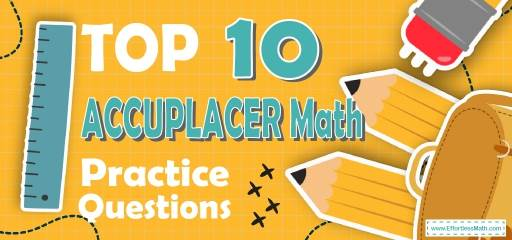# Top 10 ACCUPLACER Math Practice QuestionsTaking the Accuplacer next generation math test in a few weeks or even a few days? The best way to prepare for your Accuplaer Math test is to work through as many Accuplaer Math practice questions as possible. Here are the top 10 Accuplaer Math practice questions to help you review the most important Accuplaer Math concepts. These Accuplaer Math practice questions are designed to cover mathematics concepts and topics that are found on the actual test. The questions have been fully updated to reflect the latest 2022 Accuplaer guidelines. Answers and full explanations are provided at the end of the post.

Start your Accuplaer Math test prep journey right now with this sample Accuplaer Math questions.

## ACCUPLACER Math Practice Questions

1- $$(x + 7) (x + 5) =$$?

A. $$x^2 + 12x + 12$$

B. $$2x + 12x + 12$$

C. $$x^2 + 35x + 12$$

D. $$x^2 + 12x + 35$$

2- If $$x$$ is a positive integer divisible by 6, and $$x < 60$$, what is the greatest possible value of $$x$$?

A. 54

B. 48

C. 36

D. 59

3- $$x^2 – 81 = 0$$ , $$x$$ could equal to:

A. 6

B. 9

C. 12

D. 15

4- If $$a = 8$$, what is the value of $$b$$ in this equation?
$$b = \frac{a^2}{4} + 4$$

A. 24

B. 22

C. 20

D. 28

5- If $$6.5 < x ≤ 9.0$$, then $$x$$ cannot be equal to:

A. 6.5

B. 9

C. 7.2

D. 7.5

6- What is the area of an isosceles right triangle that has one leg that measures $$6 cm$$?

A.$$18 cm$$

B. $$36 cm$$

C. $$6 \sqrt2$$ $$cm$$

D. $$72 cm$$

7- Which of the following expressions is equivalent to $$10 – \frac{2}{3} x ≥ 12$$

A. $$x ≥ –3$$

B. $$x ≤ –3$$

C. $$x ≥ 24 \frac{1}{3}$$

D. $$x ≤ 24 \frac{1}{3}$$

8- Which of the following is a factor of both $$x^2 – 2x – 8$$ and $$x^2 – 6x + 8$$?

A. $$(x – 4)$$

B. $$(x +4)$$

C. $$(x – 2)$$

D. $$(x +2)$$

9- $$\frac{1}{6b^2} + \frac{1}{6b} = \frac{1}{b^2}$$ then $$b =$$?

A. $$-\frac{16}{15}$$

B. 5

C. $$-\frac{15}{16}$$

D. 8

10- If two angles in a triangle measure 53 degrees and 45 degrees, what is the value of the third angle?

A. 8 degrees

B. 42 degrees

C. 82 degrees

D. 98 degrees

## Best ACCUPLACER Math Prep Resource for 2022

1- D
Use FOIL (First, Out, In, Last)
$$(x + 7) (x + 5) = x^2 + 5x + 7x + 35 = x^2 + 12x + 35$$

2- D
$$\frac{54}{6}=\frac{27}{3}=9$$
$$\frac{48}{6}=\frac{24}{3}=8$$
$$\frac{36}{6}=\frac{18}{3}=6$$
$$\frac{59}{6}=\frac{59}{6}$$
59 is prime number

3- B
$$x^2 – 81 = 0 ⇒ x^2 = 81 ⇒ x = 9$$

4- C
If $$a = 8$$ then $$b =\frac{8^2}{4} + 4 ⇒ b = \frac{64}{4} + 4 ⇒b = 16 + 4 = 20$$

5- A
If $$6.5 < x ≤ 9.0$$, then x cannot be equal to 6.5

6- A
$$a = 6⇒$$ area of triangle is $$=\frac{1}{2}$$$$(6×6) = \frac{36}{2} =18$$ cm

7- B
Simplify:
$$10 – \frac{2}{3} x ≥ 12$$ ⇒$$– \frac{2}{3} x ≥ 2$$ ⇒ $$– x ≥ 3$$ ⇒ $$x ≤ – 3$$

8- A
Factor each trinomial $$x^2 – 2x – 8$$ and $$x^2 – 6x + 8$$
$$x^2 – 2x – 8 ⇒ (x – 4)(x + 2)$$
$$x^2 – 6x + 8 ⇒ (x – 2)(x – 4)$$

9- B
$$\frac{1 + b}{6 b^2} = \frac{1}{b^2}$$⇒$$(b≠0) b^2+b^3=6b^2⇒b^3-5b^2=0⇒b^2 (b-5)=0⇒b-5=0⇒b=5$$

10- C
$$53^\circ + 45^\circ = 98^\circ$$
$$180^\circ – 98^\circ = 82^\circ$$
The value of the third angle is $$82^\circ$$.

Looking for the best resource to help you succeed on the ACCUPLACER Math test?

## The Best Books to Ace the ACCUPLACER Math Test

### What people say about "Top 10 ACCUPLACER Math Practice Questions - Effortless Math: We Help Students Learn to LOVE Mathematics"?

No one replied yet.

X
52% OFF

Limited time only!

Save Over 52%

SAVE $40 It was$76.99 now it is \$36.99